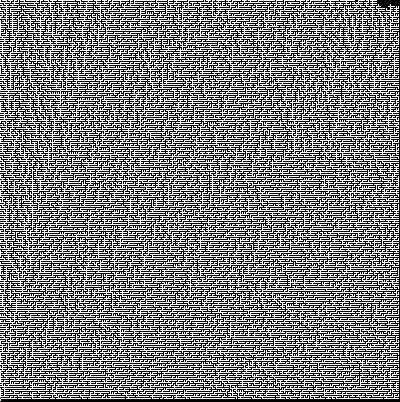# hxpctf17: irrgarten

irrgarten was a challenge for 100 points in this year's hxpctf:

Be ready for some long running in the irrgarten.

One was provided with the following initial command:

```dig -t txt -p53535 @35.198.105.104 950ae439-d534-4b0c-8722-9ddcb97a50f6.maze.ctf.link
```

Which provided the hint "try down.<domain>" in its TXT record. Resolving down.950ae439-d534-4b0c-8722-9ddcb97a50f6.maze.ctf.link yielded a CNAME to another dns name on which down.<domain> yielded yet another domain name.

Next, we tried right.<domain>, left.<domain> and up.<domain> and detected that they yielded results on some domain names as well. It seemed that the DNS records where encoding a maze.

Therefore, we wrote a small script which tried all directions on all nodes using a breadth-first search:

```#!/usr/bin/env python3
import re
import sys
from subprocess import check_output
import pickle
from concurrent.futures import ThreadPoolExecutor

RECORD_PATTERN = re.compile(r'IN (?P<record_type>.*?)\s(?P<record_value>.+?)\n')

global known
global queue
with open('cache6', 'rb') as fdes:
known, queue = pickle.load(fdes)

def save():
global known
global queue
with open('cache5', 'wb') as fdes:
pickle.dump([known, queue], fdes)

def resolve(entry: str):
out = check_output([
'dig',
'-t', 'txt' ,
'-p53535',
'@35.198.105.104',
entry
]).decode()
if 'hxp' in out:
print(out)
matches = RECORD_PATTERN.finditer(out)
result = {}
for match in matches:
result[match['record_type']] = match['record_value']
if not result:
return None
if set(result.keys()) == {'CNAME'}:
return result['CNAME']
print(entry, result)
save()
input('aa')
return result.get('CNAME')

class Node:
def __init__(self, id: str):
self.id = id
self.left = None
self.right = None
self.down = None
self.up = None

def __str__(self) -> str:
return str(self.id)

def __eq__(self, other) -> bool:
return self.id == other.id

def __hash__(self) -> int:
return hash(self.id)

if __name__ == '__main__':
known = {start}
queue = [start]

nextsave = 100
while queue:
current = queue.pop(0)
with ThreadPoolExecutor(max_workers=50) as exec:
to_resolve = ['', 'up.', 'right.', 'down.', 'left.']
if current.up is not None:
to_resolve.remove('up.')
if current.right is not None:
to_resolve.remove('right.')
if current.down is not None:
to_resolve.remove('down.')
if current.left is not None:
to_resolve.remove('left.')
futures = [
(dir, exec.submit(resolve, '{}{}'.format(dir, current))) for dir in to_resolve
]
for dir, future in futures:
#new = resolve('{}{}'.format(dir, current))
new = future.result()
if new is None:
if dir == '':
continue
setattr(current, dir[:-1], False)
continue
new = Node(new)
if dir == 'up.':
new.down = current
elif dir == 'right.':
new.left = current
elif dir == 'down.':
new.up = current
elif dir == 'left.':
new.right = current
if new not in known:
print(new)
print(len(known))
queue.append(new)
if dir != '':
setattr(current, dir[:-1], new)
nextsave -= 1
if not nextsave:
save()
nextsave = 100
print('saving')
```

As some hints were provided using TXT records, we assumed that the flag might be stored in an TXT record as well. To notice such records, the script required user input for every domain name containing anything else than a single CNAME record. After having indexed approximately 35,000 nodes, the flag was indeed presented as a TXT record:

;; AUTHORITY SECTION: maze.ctf.link. 3600 IN NS ns1.ctf.link.

;; Query time: 20 msec ;; SERVER: 35.198.105.104#53535(35.198.105.104) ;; WHEN: Fri Nov 17 17:32:52 CET 2017 ;; MSG SIZE rcvd: 224

While the script was running, we wrote another script drawing an image out of the graph data collected from the DNS:

```#!/usr/bin/env python3
import sys
import pickle
from collections import defaultdict

from PIL import Image

from pwn import Node

#sys.setrecursionlimit(50000)
matrix = defaultdict(dict)
seen = set()

global known
global queue
with open('cache5', 'rb') as fdes:
known, queue = pickle.load(fdes)

def traverse(node, x, y):
if node in seen:
return
matrix[x][y] = True

# True flag
matrix[x][y] = 'flag'
if node.id and '650a98dc-fb9e-4d73-a216-82f7e10e2216.maze.ctf.link' in node.id:
# False flag
matrix[x][y] = 'falseflag'

if node.up:
traverse(node.up, x, y + 2)
matrix[x][y + 1] = True
if node.right:
traverse(node.right, x + 2, y)
matrix[x + 1][y] = True
if node.down:
traverse(node.down, x, y - 2)
matrix[x][y - 1] = True
if node.left:
traverse(node.left, x - 2, y)
matrix[x - 1][y] = True

def to_picture():
lower_bound = min(matrix.keys()), min(min(matrix[i].keys()) for i in matrix.keys())
upper_bound = max(matrix.keys()), max(max(matrix[i].keys()) for i in matrix.keys())
width = upper_bound - lower_bound + 2
height = upper_bound - lower_bound + 2
print(lower_bound)
print(upper_bound)
img = Image.new(
'RGB',
(width, height),
'black') # create a new black image
pixels = img.load() # create the pixel map
for row_no, row in matrix.items():
#row_no = width - row_no
print(row_no - lower_bound)
for col, col_data in row.items():
print(row_no - lower_bound, col - lower_bound)
pixels[row_no - lower_bound, col - lower_bound] = (255, 255, 255)
if col_data == 'flag':
pixels[row_no - lower_bound, col - lower_bound] = (0, 255, 0)
elif col_data == 'falseflag':
pixels[row_no - lower_bound, col - lower_bound] = (0, 0, 255)
pixels[- lower_bound, - 1 - lower_bound] = (0, 255, 0)
pixels[- lower_bound, - lower_bound] = (255, 0, 0)
img.save('foo.png')

if __name__ == '__main__':
for node in known:
if node.id == '950ae439-d534-4b0c-8722-9ddcb97a50f6.maze.ctf.link':
start = node
break
traverse(start, 0, 0)
print(dict(matrix))
to_picture()
```

The actual flag, the false flag (which was sent shortly before the actual flag using a TXT record as well) and the start node are marked in different colors. The drawing is mirrored horizontally.

The image looks like this (the wrong path up from the start node is due to a mistake in the first script version storing None in the set of known nodes):